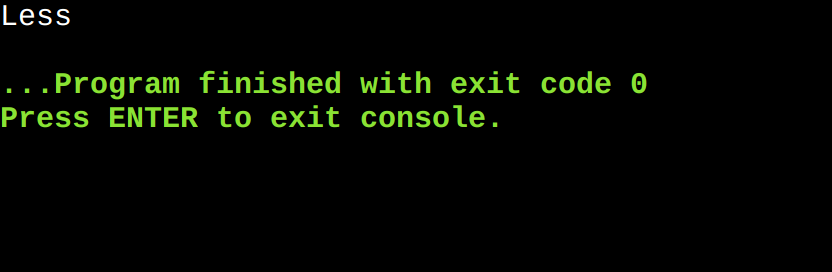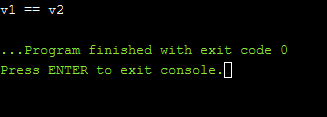Open In App

# 3-way comparison operator (Space Ship Operator) in C++ 20

The three-way comparison operator “<=>” is called a spaceship operator. The spaceship operator determines for two objects A and B whether A < B, A = B, or A > B. The spaceship operator or the compiler can auto-generate it for us. Also, a three-way comparison is a function that will give the entire relationship in one query. Traditionally, strcmp() is such a function. Given two strings it will return an integer where,

• < 0 means the first string is less
• == 0 if both are equal
• > 0 if the first string is greater.

It can give one of the three results, hence it’s a three-way comparison.

From the above table, it can be seen that the spaceship operator is a primary operator i.e., it can be reversed and corresponding secondary operators can be written in terms of it.

(A <=> B) < 0 is true if A < B
(A <=> B) > 0 is true if A > B
(A <=> B) == 0 is true if A and B are equal/equivalent.

Program 1:

Below is the implementation of the three-way comparison operator for two float variables:

## C++

 `// C++ program to illustrate the 3 way comparison``// (spaceship) operator``#include ``#include ``using` `namespace` `std;`` ` `int` `main()``{``    ``int` `x = 10;``    ``int` `y = 20;`` ` `    ``// saving the result of 3 way comparison operator``    ``auto` `res = x <=> y;`` ` `    ``// executing statements based on the above comparison``    ``if` `(res < 0)``        ``cout << ``"Less"``;``    ``else` `if` `(res > 0)``        ``cout << ``"Greater"``;``    ``else` `if` `(res == 0)``        ``cout << ``"Same"``;``    ``else``        ``cout << ``"Unordered"``;``   ` `    ``return` `0;``}`

OutputProgram 2:

Below is the implementation of the three-way comparison operator for two vectors:

## C++

 `// C++ 20 program for the illustration of the``// 3-way comparison operator for 2 vectors``#include ``using` `namespace` `std;`` ` `// Driver Code``int` `main()``{``    ``// Given vectors``    ``vector<``int``> v1{ 3, 6, 9 };``    ``vector<``int``> v2{ 3, 6, 9 };`` ` `    ``auto` `ans2 = v1 <=> v2;`` ` `    ``// If ans is less than zero``    ``if` `(ans2 < 0) {`` ` `        ``cout << ``"v1 < v2"` `<< endl;``    ``}`` ` `    ``// If ans is equal to zero``    ``else` `if` `(ans2 == 0) {`` ` `        ``cout << ``"v1 == v2"` `<< endl;``    ``}`` ` `    ``// If ans is greater than zero``    ``else` `if` `(ans2 > 0) {`` ` `        ``cout << ``"v1 > v2"` `<< endl;``    ``}`` ` `    ``return` `0;``}`

Output